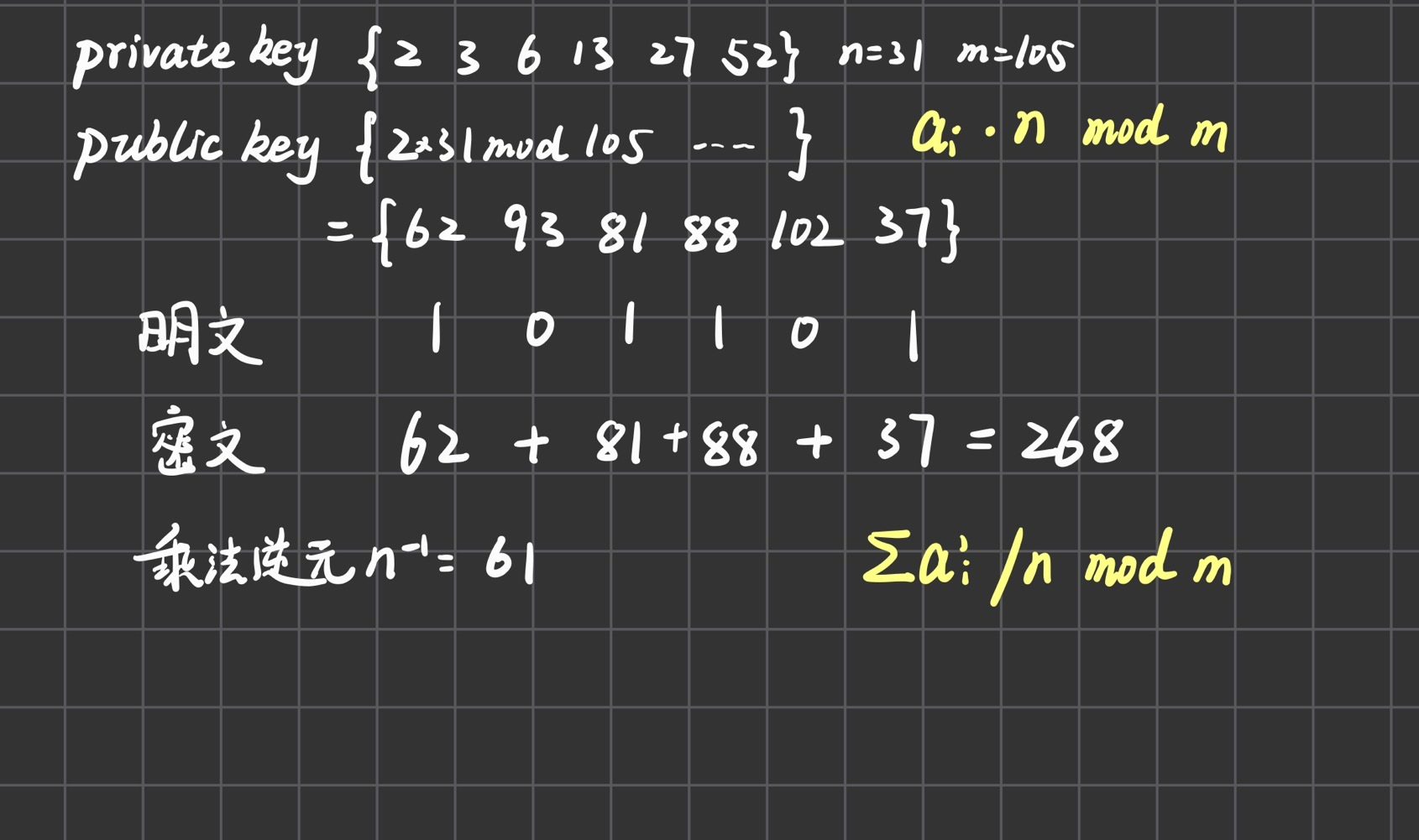# 背包密码学

Knapsack Encryption Algorithm in Cryptography - GeeksforGeeks

P问题是一类可以在多项式时间内求解的问题，NP问题是一类可以在多项式时间内验证解是否正确的问题。

NP-C或NP完全问题指的是“多项式复杂程度的非确定性问题”，所有的NP问题都可以约化到NP-C问题（即可以用NP-C问题的解法来解决NP问题，但NP-C问题的解法可能时间复杂度更高）。

1. 取一个递增序列作为私钥：(2 3 6 13 27 52)
2. 取一个与序列中所有数互质的整数$n$，再取一个大于所有数的整数$m$（$n=31,m=105$）
3. 计算$a_in\ mod\ m$，得到一个新序列作为公钥（62 93 81 88 102 37
4. 背包长度为6，所以二进制明文按照6bit分组，1表示存在，0表示不存在，然后计算总体积（明文101101，则公钥加密密文为268
5. 计算n的乘法逆元$nn^{-1}\equiv1(mod\ 105)$。（$n^{-1}=61$）
6. 计算$密文\times n^{-1}\ mod\ m$，然后解背包问题得到明文。（73=52+13+6+2(101101)